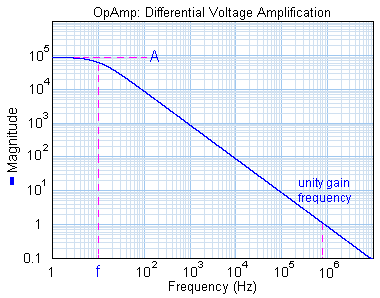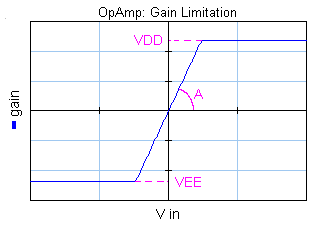﻿ 20-sim webhelp > Library > Iconic Diagrams > Electric > Components > opamp

# opamp

## Library

Iconic Diagrams\Electric\Components

Default

Universal

## Use

Domains: Continuous. Size: 1-D. Kind: Iconic Diagrams (Electric).

## Description - Default

This model represents an ideal operational amplifier. The input resistance is infinite and the output resistance is zero. The port p_in of the OpAmp model has separate high and low terminals:

p_in.u = p_in_high.u - p_in_low.u;

p_in_high.i = p_in_low.i = p_in.i = 0;

p_out.u = A*p_in.u;

## Interface - Default

 Ports Description p_in_high, P_in_low p_out Both terminals of the input port p_in (Electric). Output port (Electric). Causality fixed current out p_in fixed voltage out p_out Parameters A Amplification []

## Description - Universal

This model represents an operational amplifier with a first order decreasing voltage amplification.The amplification is parameterized by a DC-gain (A) and a cut-off frequency (f). This yields a unity gain frequency of:

unity gain frequency = A*f;

The gain is limited to yield an output that is between the positive (VDD) and negative (VEE) supply voltage.The input resistance is infinite and the output resistance is equal to the parameter R. The port p_in of the OpAmp model has separate high and low terminals:

p_in.u = p_in_high.u - p_in_low.u

p_in_high.i = p_in_low.i = p_in.i = 0;